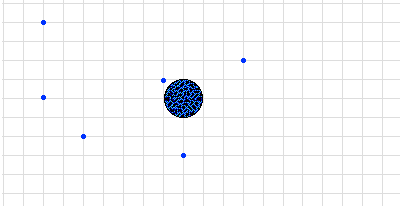## Gravitation Activity Sheet

### Purpose:

The purpose of this activity is to investigate the variables which effect the gravitational force between two objects and to investigate the effect of gravitational force upon the motion characteristics of an orbiting satellite.

### Procedure:

1. An object and the Earth are shown in the simulation window. Click and drag the object to various positions about the earth and observe the gravitational force vector. In the diagram below, draw a force vector (arrow with arrowhead) to depict the direction and relative magnitude of the force acting between the earth and the object. Note: the size of the arrow is indicative of the strength of the force.2. Is the effect between gravitational force and distance of separation (between object and Earth) an inverse or direct effect? ______________

 3. Click and drag the object to various distances from the center of the Earth in order to determine the effect of distance upon the gravitational force. Examine the effect of doubling, tripling and quadrupling the distance of separation (as measured from Earth's center). Consider the earth's surface to be a distance of one Earth-radius (1 REarth). Use the table at the right to record data for whole-number multiples of REarth. Distance Fgrav(N) 1•REarth 2•REarth 3•REarth 4•REarth 5•REarth 6•REarth 7•REarth 8•REarth 9•REarth 10•REarth

4. Return the object to a distance of approximately 3-5 Earth radii from the Earth's center. Now alter the mass of the object and the mass of the Earth. What effect - direct or inverse - do each of these alterations have upon the gravitational force between the object and the Earth?

5. Use the simulation program to answer the following questions:

If the separation distance between the object and the Earth is ... (see step #3)

... increased by a factor of 2, then the Fgrav is ___________ by a factor of _____.

... increased by a factor of 3, then the Fgrav is ___________ by a factor of _____.

... increased by a factor of 4, then the Fgrav is ___________ by a factor of _____.

If the mass of the object is ... (see step #4)

... increased by a factor of 2, then the Fgrav is increased by a factor of _____.

... increased by a factor of 3, then the Fgrav is ___________ by a factor of _____.

... decreased by a factor of 4, then the Fgrav is ___________ by a factor of _____.

If the mass of the Earth is ... (see step #4)

... increased by a factor of 2, then the Fgrav is increased by a factor of _____.

... increased by a factor of 3, then the Fgrav is ___________ by a factor of _____.

... decreased by a factor of 4, then the Fgrav is ___________ by a factor of _____.

### Summary Statement:

Summarize your findings from this lab.

Do the Gravitation Activity.